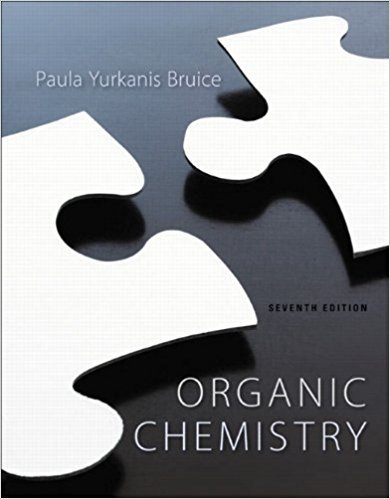×

×

Textbooks / Chemistry / Organic Chemistry 7

# Organic Chemistry 7th Edition - Solutions by Chapter## Full solutions for Organic Chemistry | 7th Edition

ISBN: 9781269406772Organic Chemistry | 7th Edition - Solutions by Chapter

Solutions by Chapter
4 5 0 304 Reviews
##### ISBN: 9781269406772

Organic Chemistry was written by and is associated to the ISBN: 9781269406772. This textbook survival guide was created for the textbook: Organic Chemistry, edition: 7. The full step-by-step solution to problem in Organic Chemistry were answered by , our top Chemistry solution expert on 09/04/17, 10:07PM. This expansive textbook survival guide covers the following chapters: 30. Since problems from 30 chapters in Organic Chemistry have been answered, more than 485566 students have viewed full step-by-step answer.

Key Chemistry Terms and definitions covered in this textbook
• absolute zero.

Theoretically the lowest attainable temperature. (5.3)

• Absorbance (A)

A quantitative measure of the extent to which a compound absorbs radiation of a particular wavelength. A 5 log (I0/I ) where I0 is the incident radiation and I is the transmitted radiation

• alcohol.

An organic compound containing the hydroxyl group —OH. (24.4)

• asymmetric hydrogenation

The addition of H2 across only one face of a p bond.

• basic oxide (basic anhydride)

An oxide that either reacts with water to form a base or reacts with an acid to form a salt and water. (Section 22.5)

• Bond dissociation enthalpy

The amount of energy required to break a bond into two radicals in the gas phase at 25°C, A!B !> A• 1 •B

• Brønsted–Lowry base

A substance (molecule or ion) that acts as a proton acceptor. (Section 16.2)

• crossed Claisen condensation

A Claisen condensation reaction that occurs between different partners.

• endergonic

Any process with a positive DG.

• kinetic control

A reaction for which the product distribution is determined by the relative rates at which the products are formed.

• law of definite proportions

A law that states that the elemental composition of a pure substance is always the same, regardless of its source; also called the law of constant composition. (Section 1.2)

• Lewis acid

A compound capable offunctioning as an electron pair acceptor.

• meta

On an aromatic ring, the C3 position.

• nitrosonium ion

The NO+ ion, which is formed when NaNO2 is treated with HCl.

• nucleon

A particle found in the nucleus of an atom. (Section 21.1)

• physiological pH

The pH of blood (approximately 7.3).

• Planck constant (h)

The constant that relates the energy and frequency of a photon, E = hn. Its value is 6.626 * 10-34 J@s. (Section 6.2)

• precipitate

An insoluble substance that forms in, and separates from, a solution. (Section 4.2)

• sp Hybrid orbitaL

A hybrid atomic orbital formed by the combination of one s atomic orbital and one 2p atomic orbital.

• Ylide

A neutral molecule with positive and negative charges on adjacent atoms